# Samacheer Kalvi 7th Maths Solutions Term 3 Chapter 1 Number System Intext Questions

Students can Download Maths Chapter 1 Number System Intext Questions and Answers, Notes Pdf, Samacheer Kalvi 7th Maths Book Solutions Guide Pdf helps you to revise the complete Tamilnadu State Board New Syllabus and score more marks in your examinations.

## Tamilnadu Samacheer Kalvi 7th Maths Solutions Term 3 Chapter 1 Number System Intext Questions

Exercise 1.1
Try These (Text book Page No. 1)

Question 1.
Represent the fraction $$\frac { 1 }{ 4 }$$ in decimal form
Solution:
$$\frac { 1 }{ 4 }$$ = $$\frac{1 \times 25}{4 \times 25}$$ = $$\frac { 25 }{ 100 }$$ = 0.25

Question 2.
What is the place value of 5 in 63.257.
Solution:
Place value of 5 in 63.257 is 5 hundredths (Hundreth place)Question 3.
Identify the digit in the tenth place of 75.036.
Solution:
0

Question 4.
Express the decimal number 3.75 as a fraction.
Solution:
3.75 = $$\frac { 375 }{ 100 }$$ = $$\frac { 15 }{ 4 }$$

Question 5.
Write the decimal number for the fraction 5 $$\frac { 1 }{ 5 }$$
Solution:
5 $$\frac { 1 }{ 5 }$$ = $$\frac { 26 }{ 5 }$$ = $$\frac{26 \times 2}{5 \times 2}$$ = $$\frac { 52 }{ 10 }$$ = 5.2

Question 6.
Identify the biggest number : 0.567 and 0.576.
Solution:
Comparing the digits of 0.567 and 0.576 from left to right, we have the tenths place same comparing the hundredths place 7 > 6.
⇒ 0.576 > 0.567Question 7.
Compare 3.30 and 3.03 and identify the smaller number.
Solution:
The whole number is equal in both the numbers.
Now comparing the tenths place we have 3 > 0
⇒ 3.03 < 3.30 Smaller number is 3.03

Question 8.
Put the appropriate sign (<, >, =). 2.57 [ ] 2.570
Solution:
2.57 [=] 2.570

Question 9.
Arrange the following decimal numbers in ascending order.
5.14, 5.41, 1.54, 1.45, 4.15, 4.51.
Solution:
Comparing the numbers from left to right. Ascending order : 1.45, 1.54, 4.15, 4.51, 5.14, 5.41

Exercise 1.2
Try These (Text book Page No. 6)

Question 1.
Find the following using grid models:
(i) 0.83 + 0.04
(ii) 0.35 – 0.09
Solution:
(i) 0.83 + 0.04
0.83 = $$\frac { 83 }{ 100 }$$ and 0.04 = $$\frac { 4 }{ 100 }$$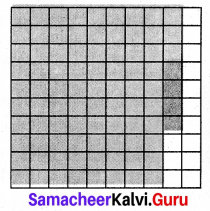0.83 and 0.04
The sum is the total shaded region.
S = 0.83 + 0.04 = 0.87

(ii) 0.35 – 0.09
0.35 = $$\frac { 35 }{ 100 }$$ and 0.09 = $$\frac { 9 }{ 100 }$$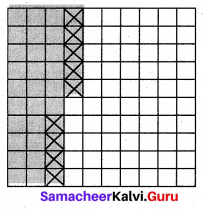Shading the regions 0.35 by shading 35 boxes out of 100. Striking off 9 boxes out of 35 shaded boxes to subtract 0.09 from 0.35.
The left over shaded boxes represent the required value.
∴ 0.35 – 0.09 = 0.26Try These (Text book Page No. 7)

Question 1.
Using the area models solve the following
(i) 1.2 + 3.5
(ii) 3.5 – 2.3
Solution:
(i) 1.2 + 3.5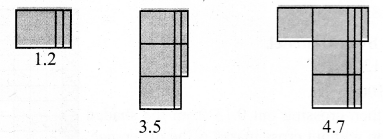Here 1.2 is represented in blue colour and 3.5 is represented in Green colour. Sum of 1.2 and 3.5 is 4.7.

(ii) 3.5 – 2.3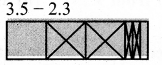Representing 3.5 using 3 squares and 5 rectangular strips. Crossing out 2 squares from 3 squares and 3 rectangular strips from 5 to get the difference. So 3.5 – 2.3 = 1.2.Try These (Text book Page No. 9)

Question 1.
Complete the magic square in such a way that rows, columns and diagonals give the same sum 1.5.Solution: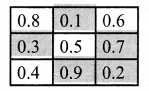Exercise 1.3
Think (Text book Page No. 13)

Question 1.
How are the products 2.1 × 3.2 and 21 × 32 alike? How are they different.
Solution:
2.1 × 3.2 = 6.72 and 21 × 32 = 672.
In both the cases the digits ambers are the same. But the place value differs.Try These (Text book Page No. 13)

Question 1.
Shade the grid to multiply 0.3 × 0.6.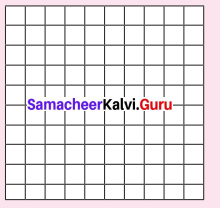Solution: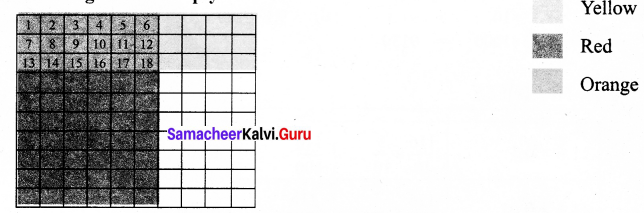3 rows of Yellow represent 0.3, 6 columns of Red colour represent 0.6 Double shaded 18 squares of orange colour represent.
∴ 0.3 × 0.6 = 0.18

Question 2.
Use the area model to multiply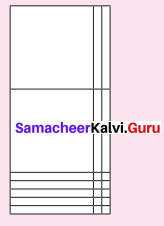Solution: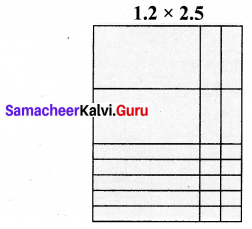Here each row contains 1 whole and 2 tenths. Each column contains 2 wholes and 5 tenths. The entire area model represents 2 wholes 9 tenths and 10 hundredths ( = 1 tenths). So 1.2 × 2.5 = 3.Try These (Text book Page No. 14)

Question 1.
Complete the following table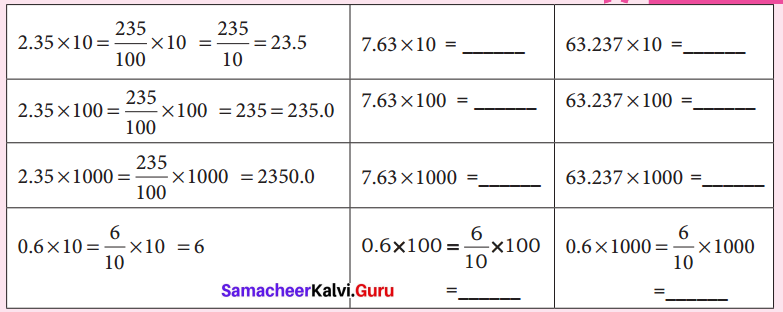Solution: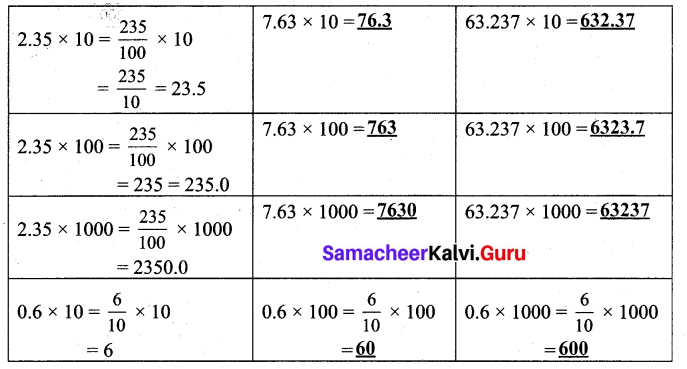Try These (Text book Page No. 15)

Question 1.
Find:

1. 9.13 × 10
2. 9.13 × 100
3. 9.13 × 1000

Solution:

1. 9.13 × 10 = 91.3
2. 9.13 × 100 = 913
3. 9.13 × 1000 = 9130Try These (Text book Page No. 16)

Question 1.
Complete the following table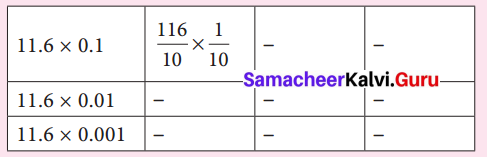Solution: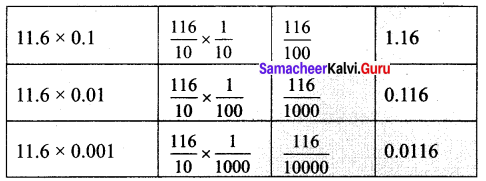Exercise 1.4
Try These (Text book Page No. 19)

Question.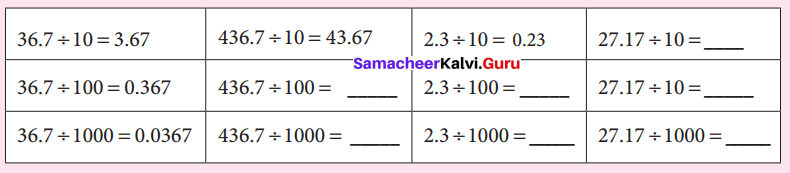Solution: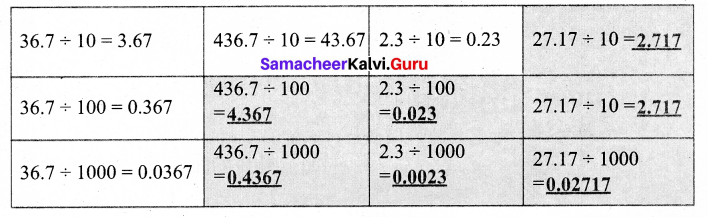Try These (Text book Page No. 19)

Question 1.
Divide the following
(i) 17.237 ÷ 10
(ii) 17.237 ÷ 100
(iii) 17.237 ÷ 1000
Solution:
(i) 17.237 ÷ 10
= $$\frac { 17237 }{ 1000 }$$ × $$\frac { 1 }{ 10 }$$
= $$\frac { 17237 }{ 1000 }$$
= 1.7237

(ii) 17.237 ÷ 100
= $$\frac { 17237 }{ 1000 }$$ × $$\frac { 1 }{ 100 }$$
= $$\frac { 17237 }{ 100000 }$$
= 0.17237

(iii) 17.237 ÷ 1000
= $$\frac { 17237 }{ 1000 }$$ × $$\frac { 1 }{ 1000 }$$
= $$\frac { 17237 }{ 1000000 }$$
= 0.017237

Try These (Text book Page No. 21)

Question 1.
Find the value of the following:
(i) 46.2 ÷ 3 = ?
(ii) 71.6 ÷ 4 = ?
(iii) 23.24 ÷ 2 = ?
(iv) 127.35 ÷ 9 = ?
(v) 47.201 ÷ 7 = ?
Solution:
(i) 46.2 ÷ 3
= $$\frac { 462 }{ 10 }$$ × $$\frac { 1 }{ 3 }$$
= $$\frac { 1 }{ 10 }$$ × $$\frac { 462 }{ 3 }$$
= $$\frac { 1 }{ 10 }$$ × 15.4
= $$\frac { 154 }{ 10 }$$
= 15.4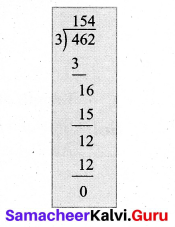(ii) 71.6 ÷ 4
= $$\frac { 716 }{ 10 }$$ × $$\frac { 1 }{ 4 }$$
= $$\frac { 1 }{ 10 }$$ × $$\frac { 716 }{ 4 }$$
= $$\frac { 1 }{ 10 }$$ × 179
= 17.9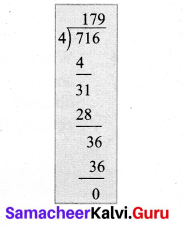(iii) 23.24 ÷ 2
= $$\frac { 2324 }{ 100 }$$ × $$\frac { 1 }{ 2 }$$
= $$\frac { 2324 }{ 2 }$$ × $$\frac { 1 }{ 100 }$$
= 1162 × $$\frac { 1 }{ 100 }$$
= $$\frac { 1162 }{ 100 }$$
= 11.62

(iv) 127.35 ÷ 9
= $$\frac { 12735 }{ 100 }$$ × $$\frac { 1 }{ 9 }$$
= $$\frac { 12735 }{ 9 }$$ × $$\frac { 1 }{ 100 }$$
= 1415 × $$\frac { 1 }{ 100 }$$
= $$\frac { 1415 }{ 100 }$$
= 14.15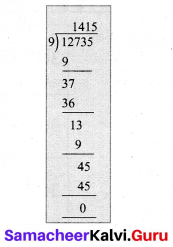(v) 47.201 ÷ 7
= $$\frac { 47201 }{ 1000 }$$ × $$\frac { 1 }{ 7 }$$
= $$\frac { 47201 }{ 7 }$$ × $$\frac { 1 }{ 1000 }$$
= 6743 × $$\frac { 1 }{ 1000 }$$
= $$\frac { 6743 }{ 1000 }$$
= 6.743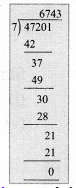Try These (Text book Page No. 22)

Question 1.
Divide the following
(i) $$\frac { 9.25 }{ 0.25 }$$
(ii) $$\frac { 8.6 }{ 4.3 }$$
(iii) $$\frac { 44.1 }{ 0.21 }$$
(iv) $$\frac { 9.6 }{ 1.2 }$$
Solution:
(i) $$\frac { 9.25 }{ 0.25 }$$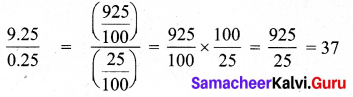(ii) $$\frac { 8.6 }{ 4.3 }$$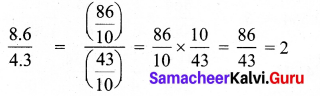(iii) $$\frac { 44.1 }{ 0.21 }$$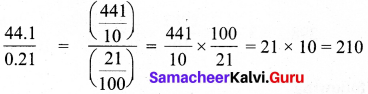(iv) $$\frac { 9.6 }{ 1.2 }$$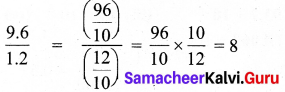Think (Text book Page No. 22)

Question 1.
The price of a tablet strip containing 30 tablets is 22.63 Then how will you find the price of each tablet?
Solution:
Price of 30 tablets = ₹ 22.63 = ₹ $$\frac { 2263 }{ 100 }$$
∴ Price of 1 tablet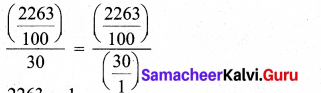= $$\frac { 2263 }{ 100 }$$ × $$\frac { 1 }{ 30 }$$
= $$\frac { 2263 }{ 30 }$$ × $$\frac { 1 }{ 100 }$$
= $$\frac { 2263 }{ 3 }$$ × $$\frac { 1 }{ 1000 }$$
= 754.33 × $$\frac { 1 }{ 1000 }$$
= $$\frac { 754.33 }{ 1000 }$$
= 0.75433
Price of each tablet is ₹ 0.7543